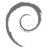# CrunchBang Linux

### SEARCH

Enter your search query in the box above ^, or use the forum search tool.

You are not logged in.

## #1 Re: Tips, Tricks & Scripts » HOW TO : using lua scripts in conky » 2014-03-03 16:37:22

@Sector11, Thank you!
I appreciate your suggestions, but but I do not want the entire code!
I desire something like this:but only the hands. How to edit my lua file to add 3 more hands?

## #2 Re: Tips, Tricks & Scripts » HOW TO : using lua scripts in conky » 2014-03-03 10:33:05

Hi to all!
How to add more watch hands with Lua? I want 3 more for:
- numeric representation of the day of the week (%u);
- two-digit day of the month (with leading zeros) (%d);
- general example for other functions.
Is it possible?
I want to make chronograph watch. The clock background will be image from Conky, and I need only from watch hands.
Here are my lua file, which is in the process of editing:

``````--[[
Clock Rings by Linux Mint (2011) reEdited by despot77

This script draws percentage meters as rings, and also draws clock hands if you want! It is fully customisable; all options are described in the script. This script is based off a combination of my clock.lua script and my rings.lua script.

IMPORTANT: if you are using the 'cpu' function, it will cause a segmentation fault if it tries to draw a ring straight away. The if statement on line 145 uses a delay to make sure that this doesn't happen. It calculates the length of the delay by the number of updates since Conky started. Generally, a value of 5s is long enough, so if you update Conky every 1s, use update_num>5 in that if statement (the default). If you only update Conky every 2s, you should change it to update_num>3; conversely if you update Conky every 0.5s, you should use update_num>10. ALSO, if you change your Conky, is it best to use "killall conky; conky" to update it, otherwise the update_num will not be reset and you will get an error.

To call this script in Conky, use the following (assuming that you save this script to ~/scripts/rings.lua):
lua_draw_hook_pre clock_rings

Changelog:
+ v1.0 -- Original release (30.09.2009)
v1.1p -- Jpope edit londonali1010 (05.10.2009)
*v 2011mint -- reEdit despot77 (18.02.2011)
]]

settings_table = {
{
-- Edit this table to customise your rings.
-- You can create more rings simply by adding more elements to settings_table.
-- "name" is the type of stat to display; you can choose from 'cpu', 'memperc', 'fs_used_perc', 'battery_used_perc'.
name='time',
-- "arg" is the argument to the stat type, e.g. if in Conky you would write \${cpu cpu0}, 'cpu0' would be the argument. If you would not use an argument in the Conky variable, use ''.
arg='%I.%M',
-- "max" is the maximum value of the ring. If the Conky variable outputs a percentage, use 100.
max=12,
-- "bg_colour" is the colour of the base ring.
bg_colour=0xffffff,
-- "bg_alpha" is the alpha value of the base ring.
bg_alpha=0,
-- "fg_colour" is the colour of the indicator part of the ring.
fg_colour=0xFF6600,
-- "fg_alpha" is the alpha value of the indicator part of the ring.
fg_alpha=0,
-- "x" and "y" are the x and y coordinates of the centre of the ring, relative to the top left corner of the Conky window.
x=100, y=150,
-- "thickness" is the thickness of the ring, centred around the radius.
thickness=5,
-- "start_angle" is the starting angle of the ring, in degrees, clockwise from top. Value can be either positive or negative.
start_angle=0,
-- "end_angle" is the ending angle of the ring, in degrees, clockwise from top. Value can be either positive or negative, but must be larger than start_angle.
end_angle=360
},
{
name='time',
arg='%S',
max=60,
bg_colour=0xffffff,
bg_alpha=0.1,
fg_colour=0xFF6600,
fg_alpha=0.6,
x=100, y=150,
thickness=5,
start_angle=0,
end_angle=360
},
{
name='time',
arg='%u',
max=7,
bg_colour=0xffffff,
bg_alpha=0.1,
fg_colour=0xFF6600,
fg_alpha=0.8,
x=100, y=250,
thickness=50,
start_angle=0,
end_angle=360
},
{
name='time',
arg='%d',
max=31,
bg_colour=0xffffff,
bg_alpha=0.1,
fg_colour=0xFF6600,
fg_alpha=0.8,
x=100, y=350,
thickness=49,
start_angle=90,
end_angle=270
},
}

-- Use these settings to define the origin and extent of your clock.

clock_r=96

-- "clock_x" and "clock_y" are the coordinates of the centre of the clock, in pixels, from the top left of the Conky window.

clock_x=96
clock_y=96

show_seconds=true

require 'cairo'

function rgb_to_r_g_b(colour,alpha)
return ((colour / 0x10000) % 0x100) / 255., ((colour / 0x100) % 0x100) / 255., (colour % 0x100) / 255., alpha
end

function draw_ring(cr,t,pt)
local w,h=conky_window.width,conky_window.height

local bgc, bga, fgc, fga=pt['bg_colour'], pt['bg_alpha'], pt['fg_colour'], pt['fg_alpha']

local angle_0=sa*(2*math.pi/360)-math.pi/2
local angle_f=ea*(2*math.pi/360)-math.pi/2
local t_arc=t*(angle_f-angle_0)

-- Draw background ring

cairo_arc(cr,xc,yc,ring_r,angle_0,angle_f)
cairo_set_source_rgba(cr,rgb_to_r_g_b(bgc,bga))
cairo_set_line_width(cr,ring_w)
cairo_stroke(cr)

-- Draw indicator ring

cairo_arc(cr,xc,yc,ring_r,angle_0,angle_0+t_arc)
cairo_set_source_rgba(cr,rgb_to_r_g_b(fgc,fga))
cairo_stroke(cr)
end

function draw_clock_hands(cr,xc,yc)
local secs,mins,hours,secs_arc,mins_arc,hours_arc
local xh,yh,xm,ym,xs,ys

secs=os.date("%S")
mins=os.date("%M")
hours=os.date("%I")

secs_arc=(2*math.pi/60)*secs
mins_arc=(2*math.pi/60)*mins+secs_arc/60
hours_arc=(2*math.pi/12)*hours+mins_arc/12

-- Draw hour hand

xh=xc+0.7*clock_r*math.sin(hours_arc)
yh=yc-0.7*clock_r*math.cos(hours_arc)
cairo_move_to(cr,xc,yc)
cairo_line_to(cr,xh,yh)

cairo_set_line_cap(cr,CAIRO_LINE_CAP_ROUND)
cairo_set_line_width(cr,5)
cairo_set_source_rgba(cr,1.0,1.0,1.0,1.0)
cairo_stroke(cr)

-- Draw minute hand

xm=xc+clock_r*math.sin(mins_arc)
ym=yc-clock_r*math.cos(mins_arc)
cairo_move_to(cr,xc,yc)
cairo_line_to(cr,xm,ym)

cairo_set_line_width(cr,3)
cairo_stroke(cr)

-- Draw seconds hand

if show_seconds then
xs=xc+clock_r*math.sin(secs_arc)
ys=yc-clock_r*math.cos(secs_arc)
cairo_move_to(cr,xc,yc)
cairo_line_to(cr,xs,ys)

cairo_set_line_width(cr,1)
cairo_stroke(cr)
end
end

function conky_clock_rings()
local function setup_rings(cr,pt)
local str=''
local value=0

str=string.format('\${%s %s}',pt['name'],pt['arg'])
str=conky_parse(str)

value=tonumber(str)
pct=value/pt['max']

draw_ring(cr,pct,pt)
end

-- Check that Conky has been running for at least 5s

if conky_window==nil then return end
local cs=cairo_xlib_surface_create(conky_window.display,conky_window.drawable,conky_window.visual, conky_window.width,conky_window.height)

local cr=cairo_create(cs)

if update_num>5 then
for i in pairs(settings_table) do
setup_rings(cr,settings_table[i])
end
end

draw_clock_hands(cr,clock_x,clock_y)
end``````

Excuse me for my bad English is not my native language!